#### Taylor Series (Linearization)

The Taylor series (linearization) method is the most commonly used method to estimate the covariance matrix of the regression coefficients for complex survey data. It is the default variance estimation method used by PROC SURVEYLOGISTIC.

Using the notation described in the section Notation, the estimated covariance matrix of model parameters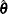by the Taylor series method is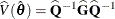where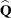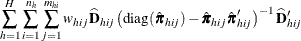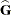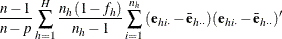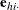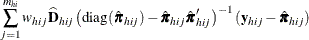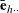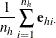and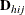is the matrix of partial derivatives of the link function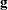with respect toand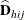and the response probabilities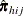are evaluated at.

If you specify the TECHNIQUE=NEWTON option in the MODEL statement to request the Newton-Raphson algorithm, the matrixis replaced by the negative (expected) Hessian matrix when the estimated covariance matrix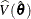is computed.